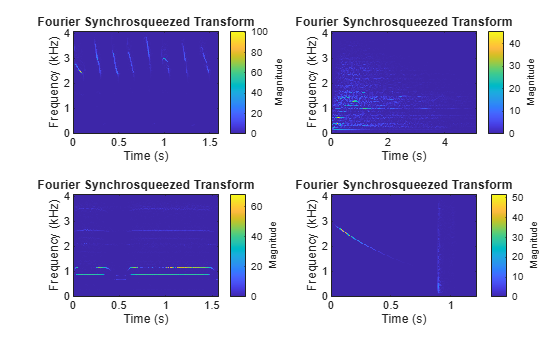## Syntax

``data = readall(sds)``

## Description

example

````data = readall(sds)` reads all signal data from the datastore `sds`.```

## Examples

collapse all

Specify the path to four signals included with MATLAB®. The signals are recordings of a bird chirp, a gong, a train, and a splat. All signals are sampled at 8192 Hz.

```folder = fullfile(matlabroot,'toolbox','matlab','audiovideo', ... ["chirp.mat","gong.mat","train.mat","splat.mat"]); fs = 8192; sds = signalDatastore(folder,'SampleRate',fs);```

Read the data in the first variables of all the files in the datastore and plot the Fourier synchrosqueezed transform of each signal.

```data = readall(sds); tiledlayout('flow') for i = 1:length(data) nexttile fsst(data{i},fs,'yaxis') end```## Input Arguments

collapse all

Signal datastore, specified as a `signalDatastore` object.

## Output Arguments

collapse all

All signals in the signal datastore, returned as a cell array. Each cell of `data` contains signals from a file or a member. Use the `ReadOutputOrientation` property of the datastore to control the orientation of `data` as either a column array or a row array.

## Tips

When reading file data, because this function reads all the data in the files at once, you may run out of memory if your dataset is large.

## Version History

Introduced in R2020a